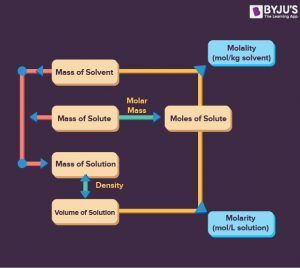Checkout JEE MAINS 2022 Question Paper Analysis : Checkout JEE MAINS 2022 Question Paper Analysis :

# Why is Molarity Useful

Concentrations expressed in molality are used in the analysis of vapour pressure and temperature changes-related solution properties. Molality is chosen because its value, with variations in temperature, does not change. On the other hand, the volume of a solution is strongly temperature-dependent.

The solution molarity is equal to the solvent moles divided by the solvent mass in kilogrammes, while the solution molarity is equal to the solvent moles divided by the solution volume in litres.

Molarity (M) is one of the most common units used to quantify the concentration of a solution, representing the amount of moles of solvent per litre of solution (moles/liter). Molarity may be used to measure the solvent volume or the solute quantity.(0)(0)